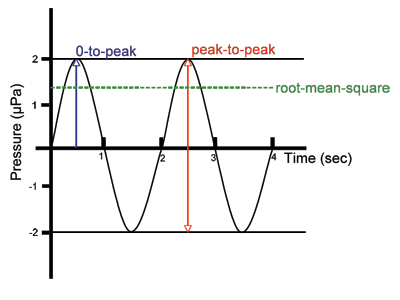# Sound Pressure Levels and Sound Exposure Levels

Regulators use sound pressure levels (SPLs) and sound exposure levels (SELs) to characterize potential effects of sound on marine animals.

Sound pressure level (SPL) is a means of characterizing the amplitude of a sound. There are several ways sound pressure can be measured. The most common of these are the root-mean-square (rms) pressure, the peak pressure, and the peak-to-peak pressure. (see: Introduction to Signal Levels)Figure 1: A simple sound wave and three common methods used to characterize the loudness of a sound signal.

Sound pressure levels are reported in units called decibels (db). It is important to tell whether the pressure being used to compute decibels is root-mean-square (rms), peak, or peak-to-peak pressure. There can be a significant difference in the sound pressure level value depending upon which measure is used. Which measure is appropriate depends on the type of signal (e.g. peak pressure or peak-to-peak pressure for impulsive signals and rms pressure for continuous signals). On the DOSITS website, underwater dB is used to indicate decibels computed using root-mean-square pressure unless otherwise indicated. For situations in which the peak pressure is used, the unit of dB peak will be indicated. If the peak-to-peak pressure is used, the unit of dB peak-peak will be indicated.

Sound exposure level (SEL) is a measure of energy that takes into account both received level and duration of exposure. SEL is a common metric since it allows sound exposures of different durations to be related to one another in terms of total acoustic energy. However, the duration of a sound event should be specified because there is no accepted standard duration over which the summation of energy is measured. SEL can be computed for a single pulse or signal, which in studies of pile driving is often referred to as single-strike SEL (SELss). It can also be computed for multiple pulses or signals to generate a value equivalent to a single exposure for the cumulative sound energy (SELcum). If the individual pulses or signals are approximately the same, then the SELcum can be estimated as SELss + 10 log10(N), where N is the number of pulses. The number of pulses should be specified, as should the total duration over which sound energy is being accumulated.

Some studies on the equal energy hypothesis have shown that SELcum can be used to predict the risk for hearing loss in mammals. This implies that sounds received at lower levels for a longer duration may have similar effects as sounds received at higher levels for a shorter duration. It is important to consider the time between individual pulses or signals since hearing can be recovered if the interval between pulses is long enough. In addition sound exposure conditions vary. Therefore a number of factors need to be considered in determining potential sound impacts.